# Volume of Rectangular Prisms: Length Width and Height

##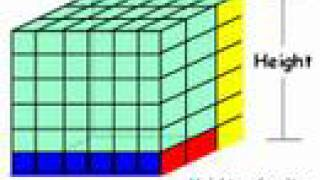By Have Fun Teaching

The Volume Song and movie teaches students how to find the volume of a solid figure. Learn how to solve and find the volume of a 3D rectangular prism shape using length times width times height.# Volume of a rectangular prism with fractional cubes

##By Khan Academy

Another way of finding the volume of a rectangular prism involves dividing it into fractional cubes finding the volume of one and then multiplying that area by the number of cubes that fit into our rectangular prism. Watch this explanation.# Volume of a Rectangular Prism

##By mrmaisonet

This video clip does a great job of visualizing volume. It also explains how the formula works in calculating the volume for any rectangular prism. To find the Volume find the B= area of base then multiply by the prisms height. V= B x h B = area of the base# Volume of Rectangular Prisms

##By Anywhere Math# Finding Volume of a rectangular prism

##By TeacherTube Math# Volume with unit cubes 2

##By Khan Academy

Another way of finding the volume of a rectangular prism involves dividing it into fractional cubes, finding the volume of one, and then multiplying that area by the number of cubes that fit into our rectangular prism.# Decompose figures to find volume

##By Khan Academy

Find the volume of an irregular 3D figure by dividing the figure into two rectangular prisms and finding the volume of each part.# Decompose figures to find volume

##By Khan Academy

Find the volume of an irregular 3D figure by dividing the figure into two rectangular prisms and finding the volume of each part.# Decompose figures to find volume

##By Khan Academy

Find the volume of an irregular 3D figure by dividing the figure into two rectangular prisms and finding the volume of each part.# Decompose figures to find volume

##By Khan Academy

Find the volume of an irregular 3D figure by dividing the figure into two rectangular prisms and finding the volume of each part.# How to Find the Volume of a Rectangular Prism and Composite figures

##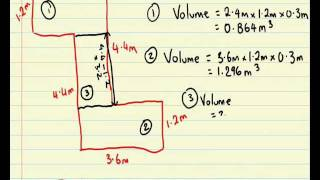By tecmath# Decompose figures to find volume

##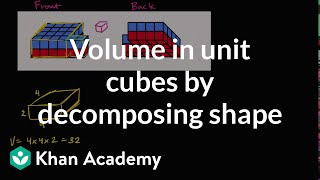By Khan Academy

Find the volume of an irregular 3D figure made up a unit cubes by dividing the figure into two rectangular prisms and finding the volume of each part.# Volume of a Prism - YourTeacher.com - Math Help

##By yourteachermathhelp

Instructor uses a white board to demonstrate how to find the volume of a rectangular prism. One example is modeled using the formula for volume and plugging in values.# Decompose figures to find volume

##By Khan Academy

Find the volume of an irregular 3D figure made up a unit cubes by dividing the figure into two rectangular prisms and finding the volume of each part.# Decompose figures to find volume

##By Khan Academy

Find the volume of an irregular 3D figure made up a unit cubes by dividing the figure into two rectangular prisms and finding the volume of each part.# Decompose figures to find volume

##By Khan Academy

Find the volume of an irregular 3D figure made up a unit cubes by dividing the figure into two rectangular prisms and finding the volume of each part.# Volume with fractions

##By Khan Academy

Learn how to find the volume of a rectangular prism that has fractional side lengths. The prism in this video has dimensions 3/5 by 1 1/6 by 3/7.# What is Volume in Math? Calculate Volume of Rectangular Prisms & Cubes w/ Units - [5-8-13]

##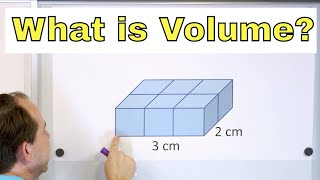By Math and Science

Quality Math And Science Videos that feature step-by-step example problems!# Inverse Variation | MathHelp.com

##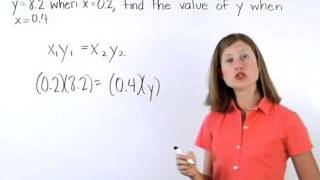By MathHelp.com

This lesson covers the surface area of a pyramid. Students learn the definition of a prism, as well as the following vocabulary words related to prisms: base, base edge, lateral edge, lateral face, and altitude (height). Students also learn the formulas for the lateral area, total area, and volume of a right prism, and are asked to solve problems using these formulas. Note that the prisms in this lesson are primarily rectangular prisms -- prisms whose bases are rectangles.# Volume of Cubes ex 2

##By sedl

Find the volume of a right rectangular prism with fractional edge lengths by packing it with unit cubes of the appropriate unit fraction edge lengths. This video is somewhat long but very clearly explains how to solve this problem.